`Redis` 中的有序集合（`Sorted Set`）就是用跳表来实现的。如果你有一定基础，应该知道红黑树也可以实现快速的插入、删除和查找操作。`Redis` 为什么会选择用跳表来实现有序集合呢？ 为什么不用红黑树呢？

## 如何理解跳表？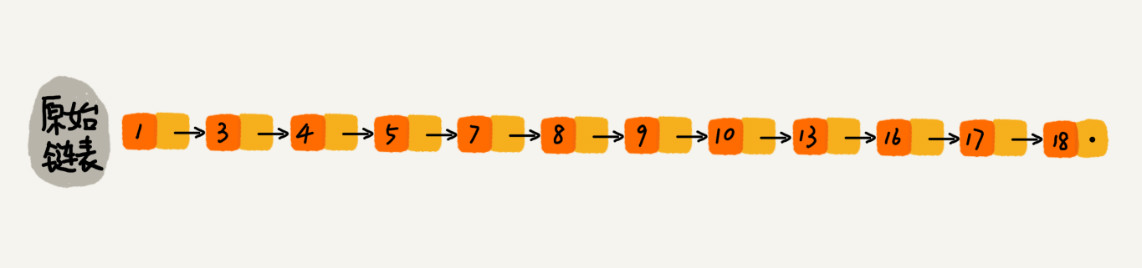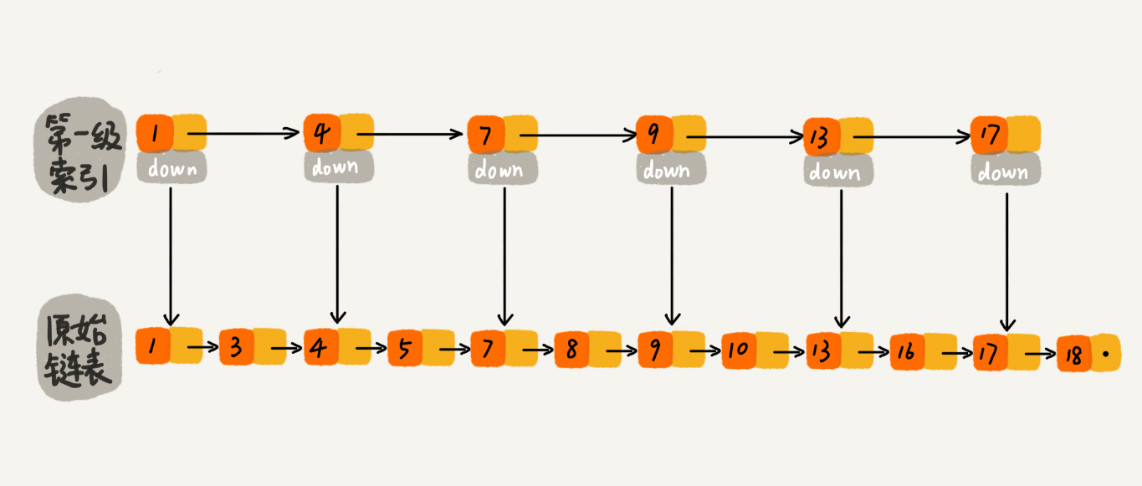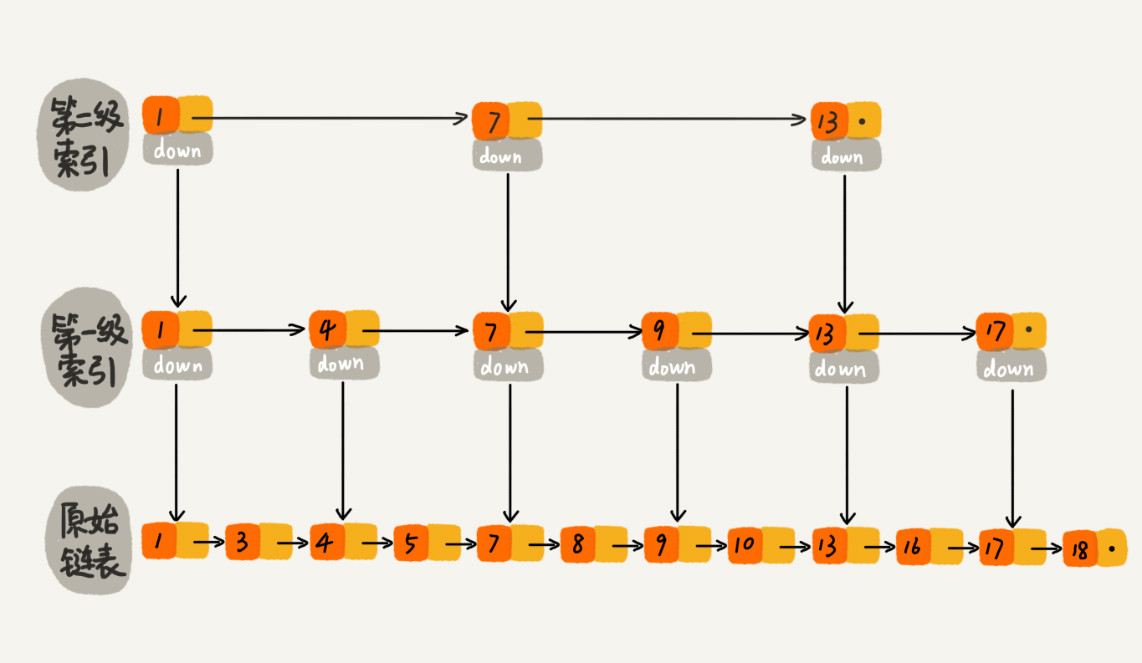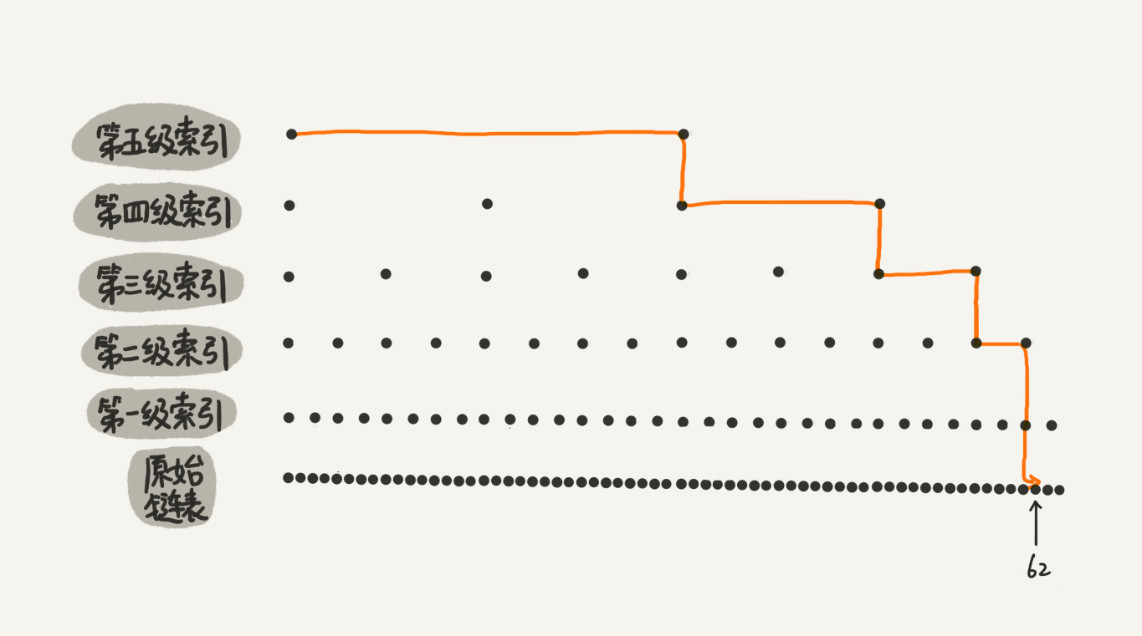## 用跳表查询到底有多快？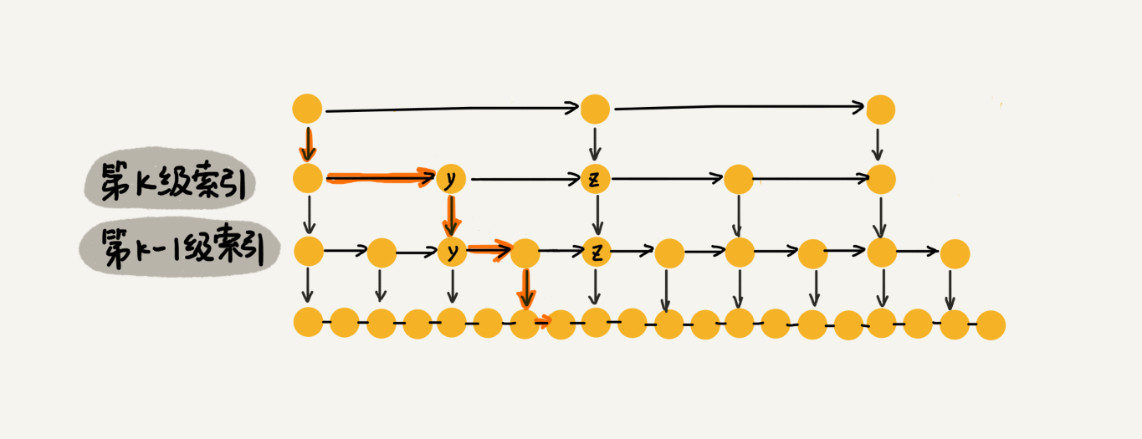## 跳表是不是很浪费内存？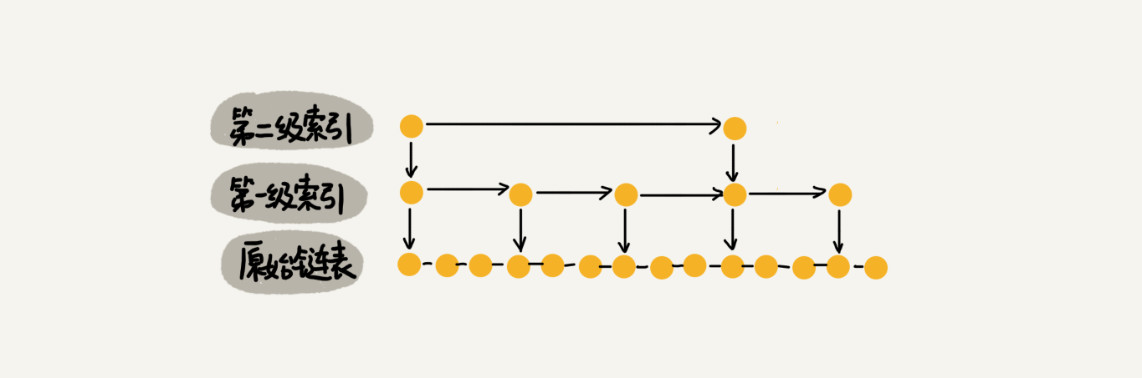## 高效的动态插入和删除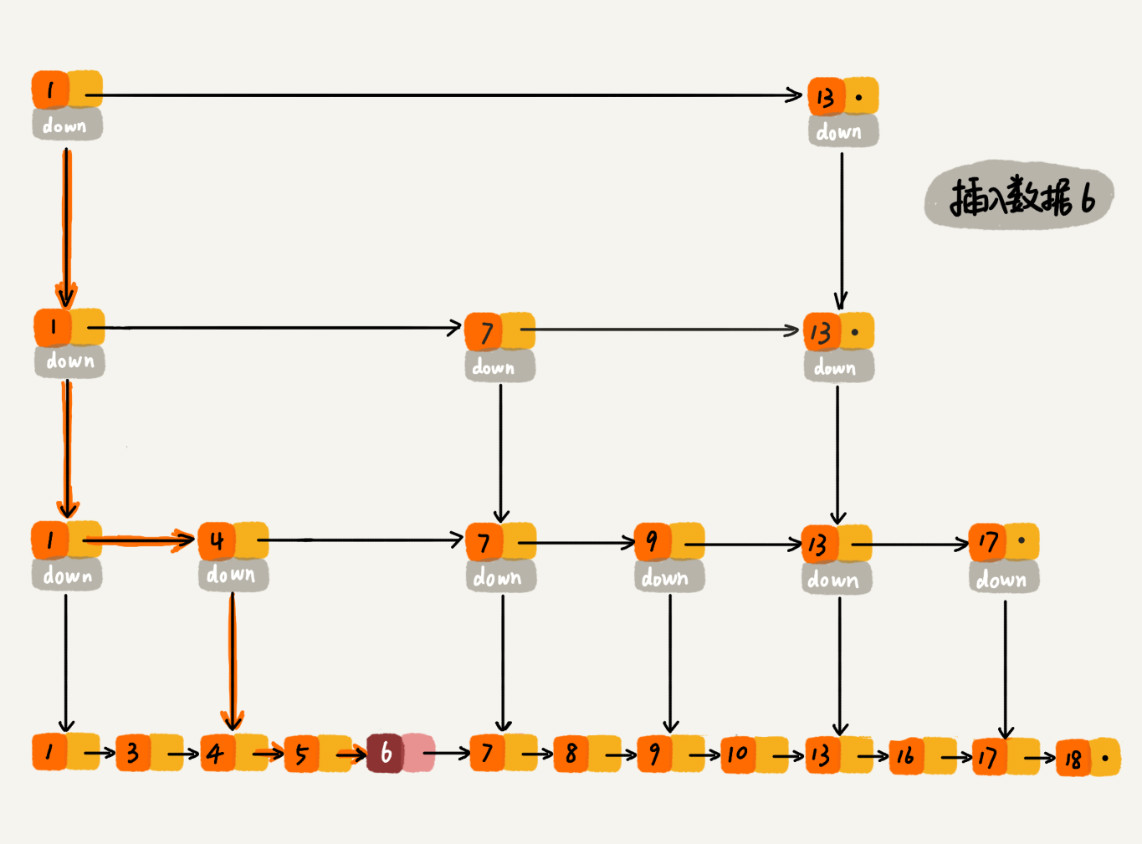## 跳表索引动态更新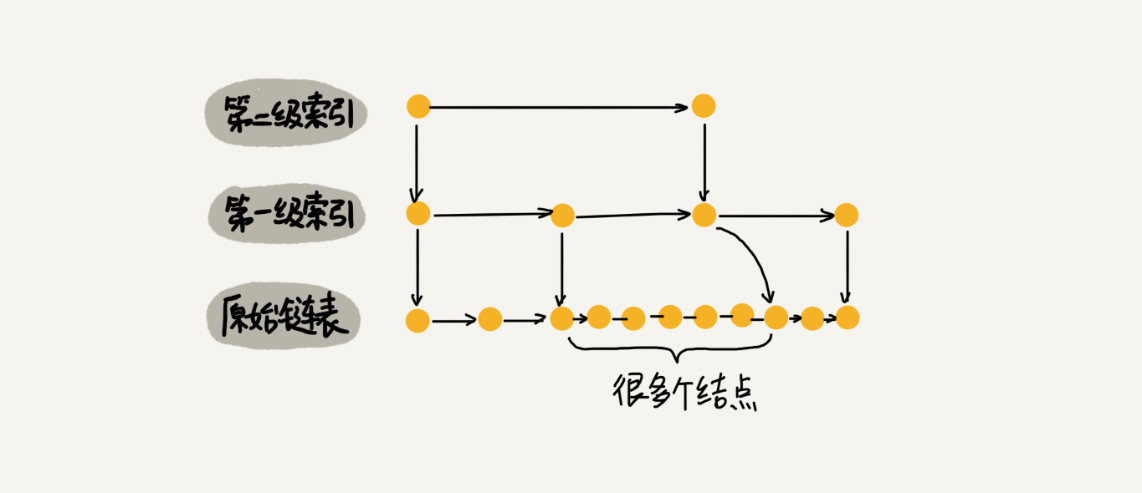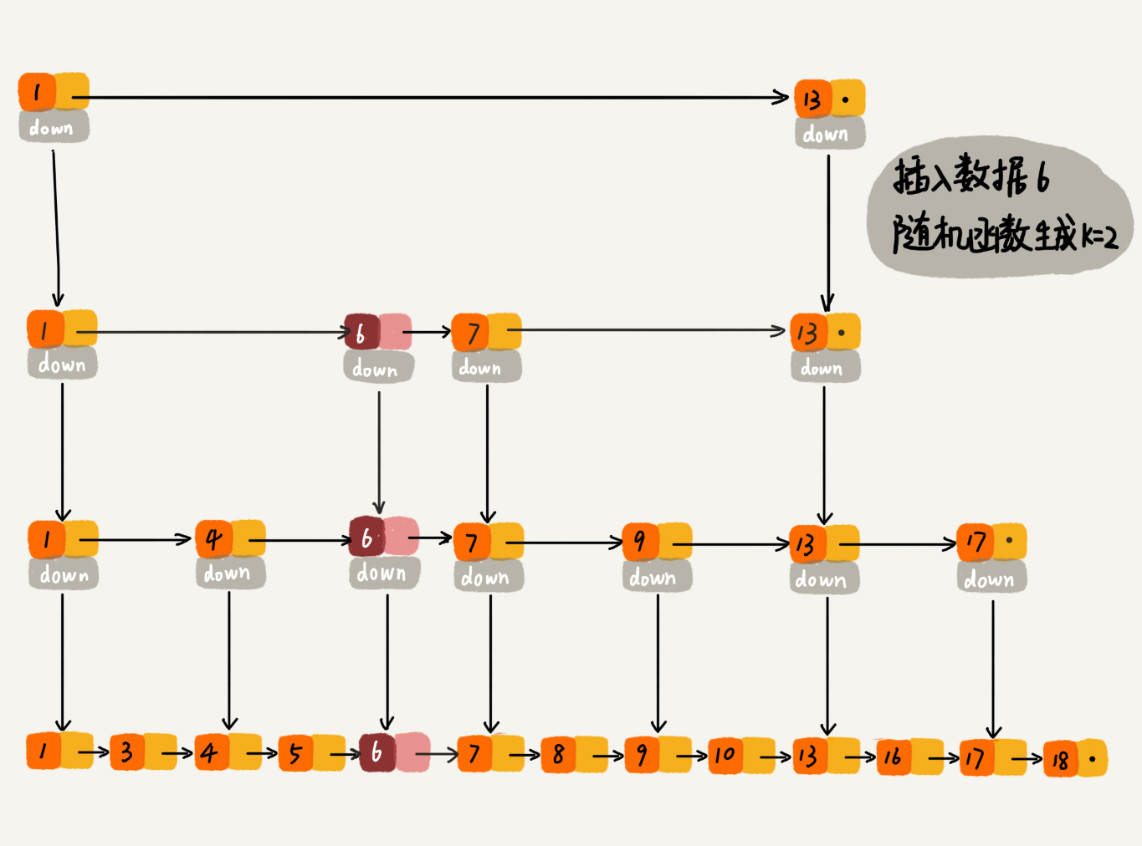## 思考

• 为什么 `Redis` 要用跳表来实现有序集合，而不是红黑树？

`Redis` 中的有序集合是通过跳表来实现的，严格点讲，其实还用到了散列表。
如果你去查看 `Redis` 的开发手册，就会发现，`Redis` 中的有序集合支持的核心操作主要有下面这几个：

• 插入一个数据；
• 删除一个数据；
• 查找一个数据；
• 按照区间查找数据（比如查找值在 `[100, 356]` 之间的数据）；
• 迭代输出有序序列。

其中，插入、删除、查找以及迭代输出有序序列这几个操作，红黑树也可以完成，时间复杂度跟跳表是一样的。但是，按照区间来查找数据这个操作，红黑树的效率没有跳表高。

对于按照区间查找数据这个操作，跳表可以做到 \$O(logn)\$ 的时间复杂度定位区间的起点，然后在原始链表中顺序往后遍历就可以了。这样做非常高效。

当然，`Redis` 之所以用跳表来实现有序集合，还有其他原因，比如，跳表更容易代码实现。虽然跳表的实现也不简单，但比起红黑树来说还是好懂、好写多了，而简单就意味着可读性好，不容易出错。还有，跳表更加灵活，它可以通过改变索引构建策略，有效平衡执行效率和内存消耗。

不过，跳表也不能完全替代红黑树。因为红黑树比跳表的出现要早一些，很多编程语言中的 `Map` 类型都是通过红黑树来实现的。做业务开发的时候，直接拿来用就可以了，不用费劲自己去实现一个红黑树，但是跳表并没有一个现成的实现，所以在开发中，如果你想使用跳表，必须要自己实现。

• 如果每三个或者五个结点提取一个结点作为上级索引，对应的在跳表中查询数据的时间复杂度是多少呢？

如果每三个或者五个节点提取一个节点作为上级索引，那么对应的查询数据时间复杂度，也还是\$O(logn)\$。
假设每 `5` 个节点提取，那么最高一层有 `5` 个节点，而跳表高度为 \$log5n\$，每层最多需要查找 `5` 个节点，即 \$O(mlogn)\$ 中的 `m = 5`，最终，时间复杂度为 \$O(logn)\$。
空间复杂度也还是 \$O(logn)\$，虽然省去了一部分索引节点，但是似乎意义不大。

## 跳表代码实现

``````package skiplist;

import java.util.Random;

/**
* 跳表的一种实现方法。
* 跳表中存储的是正整数，并且存储的是不重复的。
*
*/
public class SkipList {

private static final int MAX_LEVEL = 16;

private int levelCount = 1;

private Node head = new Node();  // 带头链表

private Random r = new Random();

public Node find(int value) {
for (int i = levelCount - 1; i >= 0; --i) {
while (p.forwards[i] != null && p.forwards[i].data < value) {
p = p.forwards[i];
}
}

if (p.forwards != null && p.forwards.data == value) {
return p.forwards;
} else {
return null;
}
}

public void insert(int value) {
int level = randomLevel();
Node newNode = new Node();
newNode.data = value;
newNode.maxLevel = level;
Node update[] = new Node[level];
for (int i = 0; i < level; ++i) {
}

// record every level largest value which smaller than insert value in update[]
for (int i = level - 1; i >= 0; --i) {
while (p.forwards[i] != null && p.forwards[i].data < value) {
p = p.forwards[i];
}
update[i] = p;// use update save node in search path
}

// in search path node next node become new node forwords(next)
for (int i = 0; i < level; ++i) {
newNode.forwards[i] = update[i].forwards[i];
update[i].forwards[i] = newNode;
}

// update node hight
if (levelCount < level) levelCount = level;
}

public void delete(int value) {
Node[] update = new Node[levelCount];
for (int i = levelCount - 1; i >= 0; --i) {
while (p.forwards[i] != null && p.forwards[i].data < value) {
p = p.forwards[i];
}
update[i] = p;
}

if (p.forwards != null && p.forwards.data == value) {
for (int i = levelCount - 1; i >= 0; --i) {
if (update[i].forwards[i] != null && update[i].forwards[i].data == value) {
update[i].forwards[i] = update[i].forwards[i].forwards[i];
}
}
}
}

// 随机 level 次，如果是奇数层数 +1，防止伪随机
private int randomLevel() {
int level = 1;
for (int i = 1; i < MAX_LEVEL; ++i) {
if (r.nextInt() % 2 == 1) {
level++;
}
}

return level;
}

public void printAll() {
while (p.forwards != null) {
System.out.print(p.forwards + " ");
p = p.forwards;
}
System.out.println();
}

public class Node {
private int data = -1;
private Node forwards[] = new Node[MAX_LEVEL];
private int maxLevel = 0;

@Override
public String toString() {
StringBuilder builder = new StringBuilder();
builder.append("{ data: ");
builder.append(data);
builder.append("; levels: ");
builder.append(maxLevel);
builder.append(" }");

return builder.toString();
}
}

}``````